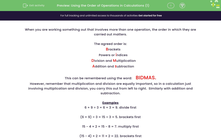# Use the Order of Operations in Calculations

In this worksheet, students will observe the order of priority in carrying out mixed operations.Key stage:  KS 3

Curriculum topic:   Number

Curriculum subtopic:   Use Conventional Notation for Priority of Operations

Difficulty level:#### Worksheet Overview

When you are working something out that involves more than one operation, the order in which it is carried out matters.

The agreed order is:

Brackets
Powers or indices
Division and Multiplication

This can be remembered using the word: BIDMAS  or BODMAS

However, remember that multiplication and division are equally important, so in a calculation just involving multiplication and division, you carry this out from left to right.  Similarly with addition and subtraction.

Examples

6 + 9 ÷ 3  The first operation to do is division because we have no brackets or indices.

6 + 3 = 9

(6 + 9) ÷ 3  This time, we have brackets, so do those first.

15 ÷ 3 = 5

15 - 4 × 2  This time, we have no brackets, indices or division, so we multiply first.

15 - 8 = 7

(15 - 4) × 2  Here, we do brackets first.

11 x 2 = 22

4 × 32 = 4 × 9 = 36 This time, we do the power first.

4 x 9 = 36

(4 × 3)2 This one needs us to do the brackets first.

122 = 144

14 - 4 + 5   This time, we have only addition and subtraction.  This means that they are equally important, so we do it left to right.

10 + 5 = 15

That's lots of examples to work through. Hopefully, they have given you a good overview of how to tackle the order of operations.

Are you ready to have a go at some questions yourself?### What is EdPlace?

We're your National Curriculum aligned online education content provider helping each child succeed in English, maths and science from year 1 to GCSE. With an EdPlace account you’ll be able to track and measure progress, helping each child achieve their best. We build confidence and attainment by personalising each child’s learning at a level that suits them.

Get started